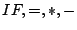Next: Steps to Eliminate Implicit Up: Using the Y-Combinator to Previous: Using the Y-Combinator to   Contents   Start

#### Introduction

The following classical example for recursive programming, the function to calculate the factorial of a natural number, is used to test the Y-combinator.

In order to simplify the procedure we use the functionsas predefined `lambda abstractions' and assume as well the availability of all natural numbers as values. To make these definitions in the Lambda Calculus would not be difficult, but it would certainly distract from our present topic.

Georg P. Loczewski 2004-03-05

Impressum und Datenschutz